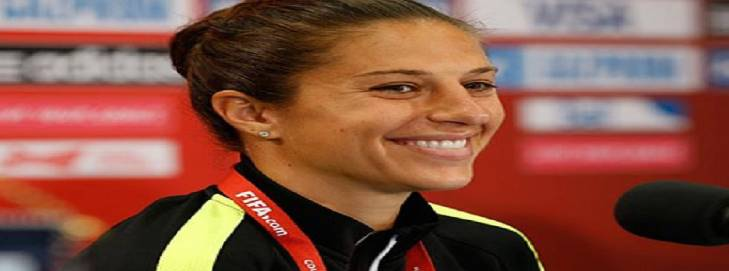# Birthday curiosities to celebrate Carli Lloyd’s new ageAziz Inan

July 15, 2019

Carli Anne Hollins (née Lloyd) is an American soccer player. She is a two-time Olympic gold medalist (2008 and 2012), two-time FIFA Women’s World Cup champion (2015 and 2019), two-time FIFA Player of the Year (2015 and 2016), and a three-time Olympian (2008, 2012, 2016) . She currently plays for Sky Blue FC of Piscataway Township, New Jersey in the National Women’s Soccer League (NWSL) and the United States women’s national soccer team (USWNT) as a midfielder.

Lloyd was born on July 16, 1982 in Delran Township, New Jersey and she will turn 37 this week. In appreciation of her outstanding performance in FIFA 2019 prepared the following numerical birthday curiosities for her:

1.      If Lloyd’s 37th birthday expressed as 7/16/2019 is split into 7, 16, and 2019, the sum of the digits of 16 equals 7, the sum of the squares of the digits of 16 yields 37, the sum of the digits of 2019 is 12 and the 12th prime number is also 37.
2.      If numbers 1 to 26 are assigned to letters A to Z, the sum of the letters numbers of Carli Lloyd add up to 111 and 111 divided by the sum of its digits equals 37 too.
3.      If 7/16/2019 is split as 716 and 2019, the sum of the squares of the digits of 716 and 2019 each equals 86. Interestingly, 86 is twice 43 and 43 equals the sum of the letter numbers of Carli.
4.      The product of the digits of 43 equals 12 and again, the 12th prime number is Lloyd’s new age, 37.
5.      Furthermore, the reverse of 86, namely 68, equals the sum of the letter numbers of Lloyd.
6.      Moreover, the sum of the letter numbers of Anne equals 34, half of 68. (Also, 3 x 4 = 12.)
7.      Additionally, the digits of Lloyd’s birthday 7/16/1982 add up to 34.
8.      Lloyd’s birth year 1982 equals twice 991, 991 is the 167th prime number, and 167 is Lloyd’s birthdate, 16 July.
9.      The sum of the digits of 716 (Lloyd’s birthdate 7/16) equals 14 and Carli Anne Lloyd is made of 14 letters.
10.     The 14th prime number is 43 (Carli).
11.     One fourth of 716 (7/16) equals 179, 179 is the 41st prime number, and the reverse of 41 is also 14.
12.     Lastly, Lloyd’s 41st birthday in 2023 expressed as 7/16/23 will be special since 23 equals 7 plus 16.  Additionally, the prime multipliers of 2023, namely 7, 17, and 17, add up to 41.

Happy 37th birthday, Carli Lloyd!

 Carli Lloyd, Wikipedia
https://en.wikipedia.org/wiki/Carli_Lloyd

Aziz Inan is a professor teaching in the electrical engineering program of the Donald P. Shiley School of Engineering at University of Portland and he can be reached at ainan@up.edu.